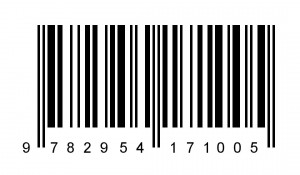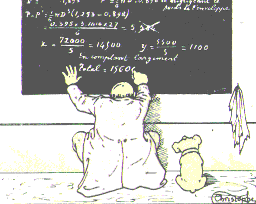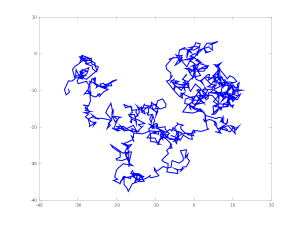# Month: March 2012Je dispense depuis deux années un cours d’initiation aux probabilités pour la préparation à l’agrégation interne de mathématiques, à l’Université Paris-Est Marne-la-Vallée. Comme souvent, j’ai pris le temps d’écrire des notes de cours, d’environ 80 pages, que je distribue à mes étudiants, et que tout le monde peut télécharger sur ma page personnelle sous forme électronique (pratique pour chercher). Ces notes n’ont rien de révolutionnaire dans leur contenu, mais expriment ma manière de voir les choses, dans le cadre du programme de l’agrégation interne (qui mériterait du reste une sérieuse refonte pour les probabilités).

Comme le soulignent mes agrégatifs, ces notes seraient beaucoup plus utiles si elles étaient transformées en livre, car seuls les livres sont autorisés par le jury le jour de l’oral du concours. La méthode traditionnelle pour transformer des notes de cours en livre consiste à contacter un éditeur, qui, bien souvent, interdira la diffusion électronique gratuite pour sauvegarder son profit. Mais pourquoi diable m’encombrer d’une telle contrainte ?

Une solution originale m’a été suggérée par courriel par un collègue distant : demander à titre personnel un numéro ISBN à l’AFNIL (gratuit). Ce service est l’analogue de celui des noms de domaines pour Internet. C’est désormais chose faite. Mes notes de cours possèdent un ISBN et constituent donc un livre électronique. Ce livre électronique est librement accessible sur ma page personnelle. Il est donc en principe utilisable le jour de l’oral par tous les candidats aux concours à partir de la session 2013 (la session 2012 est semble-t-il trop proche).

Les lecteurs qui apprécient ce livre électronique peuvent manifester leur gratitude en faisant un don directement à l’auteur via PayPal. Ce sont eux qui choisissent le montant, tandis que pour un livre classique, le prix est fixe et l’essentiel revient à l’éditeur. Cette rémunération directe de l’auteur est dans le même esprit que la rémunération directe des agriculteurs et des artistes. Elle court-circuite les marchands, qui ne produisent rien. Mais les lecteurs peuvent également choisir d’utiliser ce livre électronique sans payer, car le savoir doit rester librement accessible. Cette auto-édition et auto-diffusion est dans l’esprit de ce que prône Richard M. Stallman. Vive la révolution numérique !The next week will be very busy for me. I have to give no more than three talks in three different places. The first talk will present the beautiful and now classical Azuma-Hoeffding concentration inequality. You may take a look at the notes prepared for this talk, in French. Late update : you may also take a look at the notes of my third and last talk (in English).This short post is devoted to an intriguing random walk on the unitary group. Namely, let us fix an integer ${d\geq1}$ and pick some ${d\times d}$ unitary matrix ${U_0}$ from the unitary group ${\mathbb{U}}$. From the spectral theorem, there exists a unitary matrix ${U_1}$ in ${\mathbb{U}}$ such that ${U_1U_0U_1^*}$ is diagonal with diagonal elements in the unit circle of ${\mathbb{C}}$. This unitary diagonalization is not unique, and we may pick ${U_1}$ at random uniformly among the possibilities. This can be written as ${U_1=f(U_0,\varepsilon_1)}$ where ${\varepsilon_1}$ is a random variable. Now, we define the sequence ${{(U_n)}_{n\geq0}}$ by

$U_{n+1}=f(U_n,\varepsilon_{n+1})$

where ${{(\varepsilon_n)}_{n\geq1}}$ is an auxiliary i.i.d. sequence, chosen independent of ${U_0}$ which may be taken at random. This defines a random walk, or a Markov chain if one prefers, on the unitary group ${\mathbb{U}}$. What do we know about this chain? I have never met this chain before.

Let us say that ${Q\in\mathbb{U}}$ is a complex permutation matrix when there exists a permutation ${\sigma}$ of ${\{1,\ldots,d\}}$ and phases ${\varphi_1,\ldots,\varphi_d}$ (i.e. complex numbers of unit module) such that

$Q_{i,j}=\varphi_i\mathbf{1}_{j=\sigma(i)}$

for every ${1\leq i,j\leq d}$. This is equivalent to say that there exists a diagonal matrix ${D\in\mathbb{U}}$ and a permutation matrix ${P}$ such that ${Q=DP}$. Note that ${Q=DP=PP^{-1}DP=PD’}$ where ${D’}$ is like ${D}$. The set of complex permutation matrices is a sub-group of ${\mathbb{U}}$. If ${M}$ is a ${d\times d}$ matrix and ${Q}$ is a complex permutation matrix, then ${QM}$ is obtained from ${M}$ by permuting the rows and multiplying them by a phase (one for each).

Let us say that two elements ${U}$ and ${V}$ of ${\mathbb{U}}$ are equivalent, and we denote ${U\equiv V}$, when ${V=QU}$ for some complex permutation matrix ${Q}$. Conditional on ${U_n}$, the law of ${U_{n+1}}$ is uniform over an equivalent class for ${\equiv}$. The class of ${I_d}$ includes the commutative subgroup of ${\mathbb{U}}$ formed by diagonal elements. This class is an irreducible communication class of the chain. In contrast, if ${U_0}$ is a permutation matrix not equal to the identity then ${U_1}$ belongs to the class of a sort of bloc circulant Vandermonde matrix given by the roots of unity associated to the cycles decomposition of the permutation. On the other hand, the Haar probability measure on ${\mathbb{U}}$ is invariant for the chain. How about the spectral decomposition of the chain and its trend to the equilibrium? The convergence seems to be very fast on simulations.

Note that one may start the chain from the eigenvectors matrix of an arbitrary ${d\times d}$ random matrix ${X}$ using the unitary Schur decomposition.It is amusing to realize how a good idea may appear differently in several independent minds during the same period. Moreover, from the scientific point of view, being the first is less important than having an impact.  Regarding Brownian Motion, we find in Wikipedia:

The first person to describe the mathematics behind Brownian motion was Thorvald N. Thiele in a paper on the method of least squares published in 1880. This was followed independently by Louis Bachelier in 1900 in his PhD thesis “The theory of speculation”, in which he presented a stochastic analysis of the stock and option markets. Albert Einstein (in one of his 1905 papers) and Marian Smoluchowski (1906) brought the solution of the problem to the attention of physicists, and presented it as a way to indirectly confirm the existence of atoms and molecules.

Ironically, the microscope observation of the famous (at that time) Scottish Botanist Robert Brown regarding moving particles was anecdotal in his scientific work.

Here are some excerpts from the paper On the motion of small particles suspended in liquids at rest required by the molecular-kinetic theory of heat, by Albert Einstein, published in Annalen der Physik 17 (1905) 549–560. The original paper is in German. An English translation is available in Einstein’s miraculous year: five papers that changed the face of physics.

In this paper it will be shown that, according to the molecular kinetic theory of heat, bodies of a microscopically visible size suspended in liquids must, as a result of thermal molecular motions, perform motions of such magnitude that they can be easily observed with a microscope. It is possible that the motions to be discussed here are identical with so-called Brownian molecular motion; however, the data available to me on the latter are so imprecise that I could not form a judgment on the question.

We shall now turn to a closer examination of the disordered motions that arise from thermal molecular motion and give rise to the diffusion investigated in the last section. Obviously, we must assume that each individual particle executes a motion that is independent of the motions of all the other particles; the motions of the same particle in different time intervals must also be considered as mutually independent processes, so long as we think of these time intervals as chosen not to be too small. We now introduce a time interval τ, which is very small compared with observable time intervals but still large enough that the motions performed by a particle during two consecutive time intervals τ can be considered as mutually independent events. Suppose, now, that a total of n suspended particles is present in a liquid.

Let $f(x,t)$ be the number of particles per unit volume… we get

$$\frac{\partial f}{\partial t}=D\frac{\partial^2 f}{\partial x^2}$$
This is the well-known differential equation for diffusion, and we recognize that D is the diffusion coefficient. We have assumed that the individual particles are all referred to the same coordinate system. However, this is not necessary since the motions of the individual particles are mutually independent. … The problem, which coincides with the problem of diffusion outwards from a point (neglecting the interaction between the diffusing particles), is now completely determined mathematically; its solution is
$$f(x,t)=\frac{n}{\sqrt{4\pi D}}\frac{e^{-\frac{x^2}{4Dt}}}{\sqrt{t}}.$$
The probability distribution of the resulting displacements during an arbitrary time t is thus the same as the distribution of random errors, which was to be expected. What is important, however, is how the constant in the exponent is related to the diffusion coefficient.

Further, we found in section 4 that the mean value of the displacements of the particles in the X-direction at time t equals $\lambda_x=\sqrt{2Dt}$.

We will now calculate how large $\lambda_x$ is for one second if $N$ is taken to be $6 \times 10^{23}$ in accordance with the results of the kinetic theory of gases; water at 17° C ($k = 1.35\times 10^{-2}$) is chosen as the liquid, and the diameter of the particles is $0.001$ mm. We get $\lambda_x = 8 \times 10^{-5}$ cm $= 0.8$ micron. Therefore, the mean displacement in one minute would be about 6 microns.

Note: regarding Brownian Motion and stochastic modeling in finance, you may read the book entitled The (mis)behaviour of markets: a fractal view of risk, ruin and reward by Mandelbrot and Hudson, in particular Chapter XII entitled Ten heresies of finance.

Further reading: Sur la théorie du mouvement brownien, by Paul Langevin, 1908. Excerpt: «… J’ai pu constater tout d’abord qu’une application correcte de la méthode de M. Smoluchowski conduit à retrouver la formule de M. Einstein exactement et, de plus, qu’il est facile de donner, par une méthode toute différente, une démonstration infiniment plus simple. … »

Syntax · Style · .